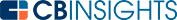# What is a Profit Margin Formula?

Profit margins are as vital to businesses as they are to financial investments and stocks. Similarly, profit margins form an essential element of accounting practices. Margins also mean different things in these three spheres: commerce, accounting, and investing.

## What are profit margin formulas used for?

Profit margins are intimately linked with investments, so it's helpful to use a collection of formulas to calculate the profits of a business or investment.

Below are a few of the main types of profit margin formulas and how to calculate them.

## Net profit margin formula

Calculating the profit margin involves a simple formula:

The net profit margin is equivalent to the revenue minus costs, divided by the revenue, which looks like this:

Net profit margin = revenue – costs / revenue

or

Net profit margin = revenue / profit x 100

One thing to remember about profit margins is to include preferred stock dividends in this calculation, but not common stocks.

When determining profit margins, context is also essential. It’s critical to use this financial ratio in conjunction with others for a broader perspective of investment health.

## Gross profit margin

Calculating the gross profit margin means determining the ratio of gross profits to sales.

The gross profit margin formula is:

Revenue less cost of goods sold (COGS) / revenue = gross profit margin

COGS is equivalent to the cost of producing the goods or services that companies offer. The gross profit margin is typically seen as an appropriate ratio to understand if the pricing of its goods is cost-effective.

## Operating profit margin

Similarly, the operating profit margin is:

Operating profit margin = operating income / revenue x 100

In other words, this financial ratio indicates the ratio between the company’s operating profits and its sales. Companies exclude certain costs such as financing and selling, general and administrative expenses (SG&A) to calculate the operating margin.

## Rate of profit or rate of return

The rate of profit is also referred to as the rate of return (RoR) and links directly to profit margins.

The formula for the RoR is:

Rate of profit = current value – original value / (original value x 100)

Investors use several measures to calculate profit earnings in relation to capital investment, also known as the stock of capital.

The RoR links to how much capital investment is required to generate a certain profit level. In other words, the RoR refers to the efficacy of the capital investment in terms of the profits generated over a specific period.

## Return on investment (ROI)

The ROI refers to the returns that an investment has or will potentially generate without accounting for its efficiency over time (the holding period).

The formula to calculate the ROI is:

Return on investment = net return on investment / (cost of investment x 100)

The ROI financial ratio compares investment gains and losses in relation to the investment's original cost. Investors use the ROI to rank single or multiple investments in conjunction with other typical cash flow measures such as the net present value (NPV) and/or the internal rate of return (IRR).

An ROI calculation is typically expressed as a percentage, which is intuitively easier to understand when ranking possible investment opportunities for decision purposes.

## Annualized return on investment

Essentially, the annualization of the popular ROI financial ratio compensates for the absence of a timeframe in the return on investment formula.

The formula for the annualized return on investment is:By annualizing the ROI, investors obtain a broader perspective of the possible gains or losses to be made in a specific timeframe.

## ﻿Investor leverage and margins

Investors can also create leverage by buying on margins. Buying on margins refers to the situation when an investor borrows money from a broker to leverage possible gains on stock shares or securities.

Leveraging margins effectively means that investors will amplify their gains or losses when stock prices rise or fall. Investors also pay broker fees when leveraging margins. Brokers will issue investors with a margin call when stock prices fall to restore the investment equity to its original position.

Profit margins characterize commerce and investments. These few basic formulas only represent the tip of the iceberg when it comes to navigating your way through the multitude of financial investments.

You may also like# www.cbinsights.com